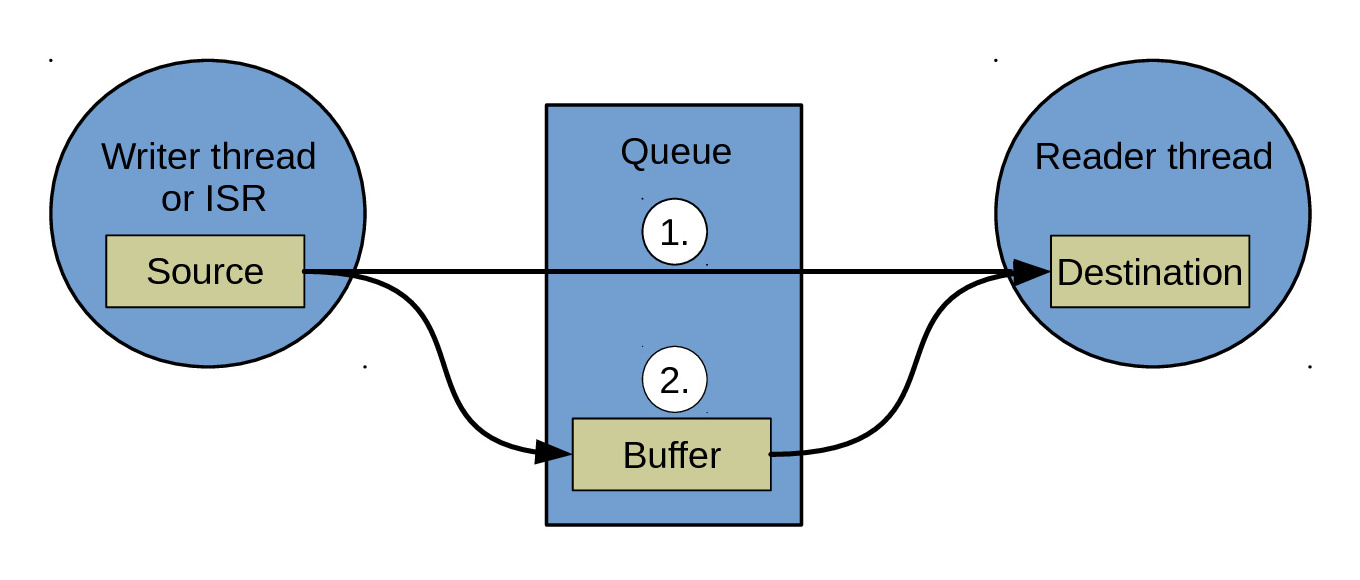# 2.4. `queue` — Queue channel¶

The most common channel is the queue. It can be either synchronous or semi-asynchronous. In the synchronous version the writing thread will block until all written data has been read by the reader. In the semi-asynchronous version the writer writes to a buffer within the queue, and only blocks all data does not fit in the buffer. The buffer size is selected by the application when initializing the queue.

The diagram below shows how two threads communicates using a queue. The writer thread writes from its source buffer to the queue. The reader thread reads from the queue to its destination buffer.The data is either copied directly from the source to the destination buffer (1. in the figure), or via the internal queue buffer (2. in the figure).

1. The reader thread is waiting for data. The writer writes from its source buffer directly to the readers’ destination buffer.
2. The reader thread is not waiting for data. The writer writes from its source buffer into the queue buffer. Later, the reader reads data from the queue buffer to its destination buffer.

Source code: src/sync/queue.h, src/sync/queue.c

Test code: tst/sync/queue/main.c

Test coverage: src/sync/queue.c

Example code: examples/queue/main.c

Defines

`QUEUE_INIT_DECL`(_name, _buf, _size) struct queue_t _name = { \ .base = { \ .read = (chan_read_fn_t)queue_read, \ .write = (chan_write_fn_t)queue_write, \ .size = (chan_size_fn_t)queue_size, \ .control = chan_control_null, \ .reader_p = NULL, \ .list_p = NULL \ }, \ .writers = { \ .head_p = NULL, \ }, \ .writer_p = NULL, \ .buffer = { \ .begin_p = _buf, \ .read_p = _buf, \ .write_p = _buf, \ .end_p = &_buf[_size], \ .size = _size \ }, \ .state = QUEUE_STATE_INITIALIZED, \ .reader = { \ .buf_p = NULL, \ .size = 0, \ .left = 0 \ } \ }

Enums

enum `queue_state_t`

Values:

`QUEUE_STATE_INITIALIZED` = 0

Queue initialized state.

`QUEUE_STATE_RUNNING`

Queue running state.

`QUEUE_STATE_STOPPED`

Queue stopped state.

Functions

int `queue_init`(struct queue_t *self_p, void *buf_p, size_t size)

Initialize given queue.

Return
zero(0) or negative error code
Parameters
• `self_p`: Queue to initialize.
• `buf_p`: Buffer.
• `size`: Size of buffer.

int `queue_start`(struct queue_t *self_p)

Start given queue. It is not required to start a queue unless it has been stopped.

Return
zero(0) or negative error code.
Parameters
• `self_p`: Queue to start.

int `queue_stop`(struct queue_t *self_p)

Stop given queue. Any ongoing read and write operations will return with the currently read/written number of bytes. Any read and write operations on a stopped queue will return zero(0).

Return
true(1) if a thread was resumed, false(0) if no thread was resumed, or negative error code.
Parameters
• `self_p`: Queue to stop.

int `queue_stop_isr`(struct queue_t *self_p)

Same as `queue_stop()` but from isr or with the system lock taken (see `sys_lock()`).

ssize_t `queue_read`(struct queue_t *self_p, void *buf_p, size_t size)

Return
Number of read bytes or negative error code.
Parameters
• `self_p`: Queue to read from.
• `buf_p`: Buffer to read to.
• `size`: Size to read.

ssize_t `queue_write`(struct queue_t *self_p, const void *buf_p, size_t size)

Write bytes to given queue. Blocks until size bytes has been written.

Return
Number of written bytes or negative error code.
Parameters
• `self_p`: Queue to write to.
• `buf_p`: Buffer to write from.
• `size`: Number of bytes to write.

ssize_t `queue_write_isr`(struct queue_t *self_p, const void *buf_p, size_t size)

Write bytes to given queue from isr or with the system lock taken (see `sys_lock()`). May write less than size bytes.

Return
Number of written bytes or negative error code.
Parameters
• `self_p`: Queue to write to.
• `buf_p`: Buffer to write from.
• `size`: Number of bytes to write.

ssize_t `queue_size`(struct queue_t *self_p)

Get the number of bytes currently stored in the queue. May return less bytes than number of bytes stored in the channel.

Return
Number of bytes in queue.
Parameters
• `self_p`: Queue.

ssize_t `queue_unused_size`(struct queue_t *self_p)

Get the number of unused bytes in the queue.

Return
Number of bytes unused in the queue.
Parameters
• `self_p`: Queue.

ssize_t `queue_unused_size_isr`(struct queue_t *self_p)

Get the number of unused bytes in the queue from isr or with the system lock taken (see `sys_lock()`).

Return
Number of bytes unused in the queue.
Parameters
• `self_p`: Queue.

struct `queue_buffer_t`

Public Members

char *`begin_p`
char *`read_p`
char *`write_p`
char *`end_p`
size_t `size`
struct `queue_writer_elem_t`

Public Members

struct thrd_prio_list_elem_t `base`
void *`buf_p`
size_t `size`
size_t `left`
struct `queue_t`

Public Members

struct chan_t `base`
struct thrd_prio_list_t `writers`
struct queue_writer_elem_t *`writer_p`
char *`buf_p`
size_t `size`
size_t `left`
`struct queue_t::@103 queue_t::reader`
struct queue_buffer_t `buffer`
queue_state_t `state`